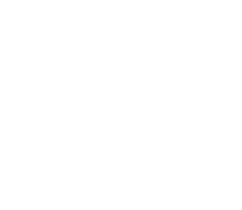# Group 5

## Mathematics

There are two different maths courses available to IB students.

Mathematics: Analysis and Approaches (HL & SL)

This course is designed for students who enjoy developing their mathematics to become fluent in the construction of mathematical arguments and develop strong skills in mathematical thinking. They will explore real and abstract applications, sometimes with technology, and will enjoy the thrill of mathematical problem solving and generalization.

Students who plan to pursue study as pure mathematicians, engineers, physical scientists, and economists or those with an interest in theoretical mathematics and analytic methods are encouraged to take this course.

Mathematics: Applications and Interpretation (SL only)

This course is for students who are interested in developing their mathematics for describing our world, modelling and solving practical problems using the power of technology. Students who take Mathematics: Applications and interpretation will be those who enjoy mathematics best when seen in a practical context.

Students who plan to pursue study as social scientists, arts, some economists, natural scientists, and students with an interest in the practical applications of mathematics through technology are encouraged to take this course.

COURSE CONTENT

All students will explore mathematical concepts in five areas of mathematical study:

• Number and Algebra
• Functions
• Geometry and Trigonometry
• Probability and Statistics
• Calculus

• Analysis and Approaches focuses on analytic methods of mathematics with an emphasis on algebra and calculus.
• Applications and Interpretation focuses on practical methods of mathematics with an emphasis on statistics and the use of technology.

ASSESSMENT

Internal

An individual investigation project               20%

External

Written examinations

(SL 2 papers, HL 3 Papers)                                 80%

REQUIREMENTS

Higher level maths: This is a demanding course for candidates with a strong background and proven ability in mathematics (a history of mostly A and some B grades).

Standard level maths: A sound background in mathematics .